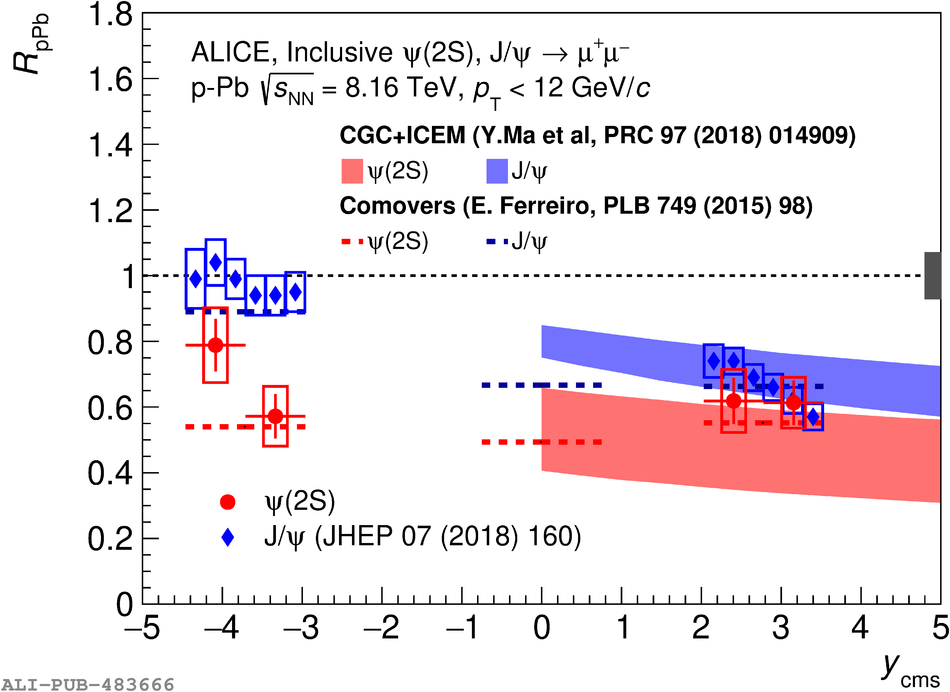# Figure 6

 The $y_{\rm cms}$-dependence of $R_{\rm{pPb}}$ for \psip and \jpsi  in p--Pb collisions at $\sqrtSnn = 8.16$ TeV. The error bars represent the statistical uncertainties, while the boxes correspond to uncorrelated systematic uncertainties and the box at $R_{\rm pPb}=1$ to correlated systematic uncertainties. The results are compared with models including initial-state effects  and coherent energy loss  (left panel), and to models which also implement final-state effects  (right panel).